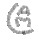Unresolved Detail In Plotted Equations

Sometimes the calculator detects that an equation is too complicated to plot perfectly in a reasonable amount of time. When this happens, the equation is plotted at lower resolution.

235 out of 261 found this helpful
Have more questions? Submit a request

•None

Also replace the x to make y=tan(x^2+2^y)............

Edited by None
•RT4832

\frac{\cos\left(x\right)}{\cos\left(y\right)}=y!

•RT4832

\left(\sin(x)+\cos(y))=\sin(x+y!\right)

•RT4832

\operatorname{mod}\left(\cos\left(\frac{\sin x}{\cos y}\right),\frac{\cos\left(y!\right)}{\cos\left(\sin\left(x^{\left(\sin y\right)}\right)\right)}\right)=0

•Lego_Master_A (LMA)

x=\cos\left(\sin\left(\tan\left(xy\right)\right)\right) is insane!!

•Ze_Mathster

I tried x! = y^y and it reponded at a rate of 1 fr/sec. So laggy.

•Ze_MathsterHello whatsit doing?! So laggy.

•f((x+y)cot(xy))=3

Edited by Yahya Abusaad
•Ze_Mathster

(sin(cos(xy))x(tan(cot((x^2)(y^(7y))))=x^2 =

Mass computerize total and utter destruction. Heck no what happened to my computer...•Ze_Mathster

Another one: sin(xy)=cot(x^y).

Hello?! This is too much.ç

••mATT C

Chances are this one is a maze.

x^{x^{\cos\left(x\right)}}=y^{y^{\sin\left(y\right)}}

•Ze_Mathster

Lesson one on how to break your computer...

•Ze_MathsterOh hell by wifi...

x^x^cos(xy^2^x^y^cot(xy))=xy

•Hbaxter9219

\frac{10}{\frac{.27}{\frac{1}{6x}}}=\frac{y}{\frac{2}{\cos\left(xy^2\right)}}  Super Amazing!!!!!

•mATT C

https://www.desmos.com/calculator/wct1vqdrgi

Planet Lag (Taking out the inequality x^2+y^2\le5 causes huge trouble)

•22yatzac

if you wait long enough it will always load the actual thing and from what ive seen most of the equations provided here are awesome

•Savion Steinmetz

Here is one
cos\left(x\right)\left(-sin^2\left(x\right)\right)=0

•00058707

I have done the unspeakable https://www.desmos.com/calculator/3tau3bg9gl

Warning, takes a while to load

Edited by 00058707
•Ze_Mathster

Do you want to crash desmos...cot(x^sech(x^y^tan(sinh(sec(xy^x^y)))))=sec(x^7xy)

•Ze_Mathster

Matt, it looks like green Jupiter

•Ze_Mathster
Edited by Ze_Mathster
•Rhu 25

8*sin(xy)=tan(y)

it will kill desmos

•mATT C

Ze_Mathster. sure enough.

•mATT C
•GlitchedGraph666

0=\frac{x^2y^2\sin\left(xy\right)+\frac{1}{x}-\frac{\pi^{yx}\tan\left(x\right)}{\csc\left(x^4\right)y}+\frac{7x+y^2}{2^xy^2}}{x\cos\left(y\right)+\frac{4x\csc\left(x^{5y}\right)\cdot4\left|x\right|}{xy\left|\frac{x}{2^3}\right|+\frac{7}{xy\tan\left(x\right)}+\pi\sqrt{\pi}}} is hyper insane

•Kestmartin

tan xy = cos xy

•Williamlambert929

\frac{\sin\left(x\left(\cos xy\right)\right)}{\tan\left(x^y+\frac{2}{y^{\cos\left(x\right)}}\right)}=y

is super weird

•RT4832

\cos\left(\operatorname{mod}\left(x,y\right)\right)=\tan\left(y\right)

it's... it's so beautiful!

•mATT C
Article is closed for comments.